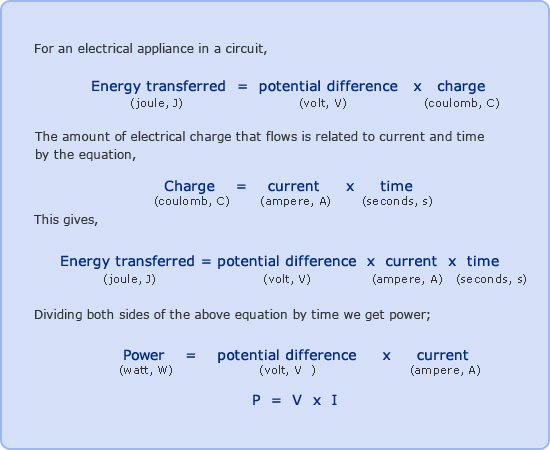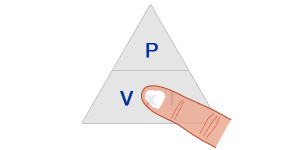## Electrical Power

Electrical appliances transform energy. The power of an electrical appliance is the rate at which it transforms energy.

Power is defined as the rate of energy transfer. It is measured in watts (W) with 1 watt being equal to 1 joule per second.### Example

An electric heater connected to the 230V mains supply draws a current of 4A. Calculate the power of the electric heater?

Potential Difference = V = 230V
Current = I = 4A
Power = P = ?

Using P = V x I

P = 230 x 4 = 920W

Most electrical appliances have their power and the potential difference of the connecting supply printed on them. With this information we can calculate the required current and the rating of the fuse required.

### Example – Fuse Rating

An electric kettle has a 2500W on a 230V main supply. What fuse should be fitted in the plug?

Power = P = 2500W
Potential Difference = V = 230V
Current = I = ?

Using P = V x I triangle,We require I so cover I on the triangle which gives

Thus,
I = P / V
I = 2500 / 230 = 10.87A

Therefore a 13A fuse should be fitted.

Remember: Fuse values are only available in 3A, 5A and 13A values

The energy transferred by an electrical appliance can be determined from the relationship

Energy transferred (joules, J)
= Power (watts, W) x Time (seconds, s)

Therefore, if an electric bulb with a power of 60W is on for 10 minutes the energy transferred as heat and light can be calculated as follows:

Power = P = 60W
Time = t = 10 mins = 10 x 60 = 600s

Energy transferred = 60 x 600 = 36000J = 36kJ